Math resources Geometry

Surface area

# Surface area

Here you will learn about surface area, including what it is and how to calculate it for prisms and pyramids.

Students will first learn about surface area as part of geometry in 6 th grade.

## What is surface area?

The surface area is the total area of all of the faces of a 3D shape. This includes prisms and pyramids. The surface area is always recorded in square units.

Prisms are 3D shapes that have a polygonal base and rectangular faces. A rectangular prism has 6 rectangular faces, including 4 rectangular lateral faces and 2 rectangular bases.

For example,

Calculate the area of each face and then add them together for the surface area of the rectangular prism.

The surface area of the prism is the sum of the areas. Add each area twice, since each rectangle appears twice in the prism:

8+8+12+12+6+6=52 \, f t^2

You can also find the surface area by multiplying each area by 2 and then adding.

(2 \times 8)+(2 \times 12)+(2 \times 6)=52 \, f t^2

Step-by-step guide: Surface area of rectangular prism

Another type of prism is a triangular prism.

A triangular prism is made up of 5 faces, including 2 triangular bases and 3 rectangular lateral faces.

For example,

Calculate the area of each face and then add them together for the surface area of the triangular prism.

Left side
lateral face
Bottom
lateral face
Right side
lateral face
Triangular
base

\begin{aligned} A&=6 \times 6.4 \\ &=38.4 \mathrm{~mm}^2 \end{aligned}

\begin{aligned} A&=6 \times 10 \\ &=60 \mathrm{~mm}^2 \end{aligned}

\begin{aligned} A&=6 \times 6.4 \\ &=38.4 \mathrm{~mm}^2 \end{aligned}

\begin{aligned} A&=\frac{1}{2} \times 10 \times 4.2 \\ &=21 \mathrm{~mm}^2 \end{aligned}

The surface area of the prism is the sum of the areas. Add the area of the triangular base twice (or you can multiply it by 2 ), since it appears twice in the prism:

37.2+60+38.4+21+21=177.6 \mathrm{~mm}^2

Step-by-step guide: Surface area of triangular prism

Step-by-step guide: Surface area of a prism

Pyramids are another type of 3D shape. A pyramid is made up of a polygonal base and triangular lateral sides.

For example,

All lateral faces (sides) of this square pyramid are congruent.

To calculate the surface area of a pyramid, calculate the area of each face of the pyramid and then add the areas together.

\text {Area of the base }=2.5 \times 2.5=6.25 \mathrm{~cm}^2

\text {Area of a triangular face }=\cfrac{1}{2} \times 2.5 \times 4=5 \mathrm{~cm}^2

Add the area of the base and the 4 congruent triangular faces:

\text {Surface area }=6.25+5+5+5+5=6.25+(4 \times 5)=26.25 \mathrm{~cm}^2

The total surface area can also be written in one equation:

​​\begin{aligned} \text {Surface area of pyramid } & =\text {Area of base }+ \text {Areas of triangular faces } \\\\ & =2.5^2+4 \times\left(\cfrac{1}{2} \, \times 2.5 \times 4\right) \\\\ & =6.25+20 \\\\ & =26.25 \mathrm{~cm}^2 \end{aligned}

Step-by-step guide: Surface area of a pyramid

### What is surface area?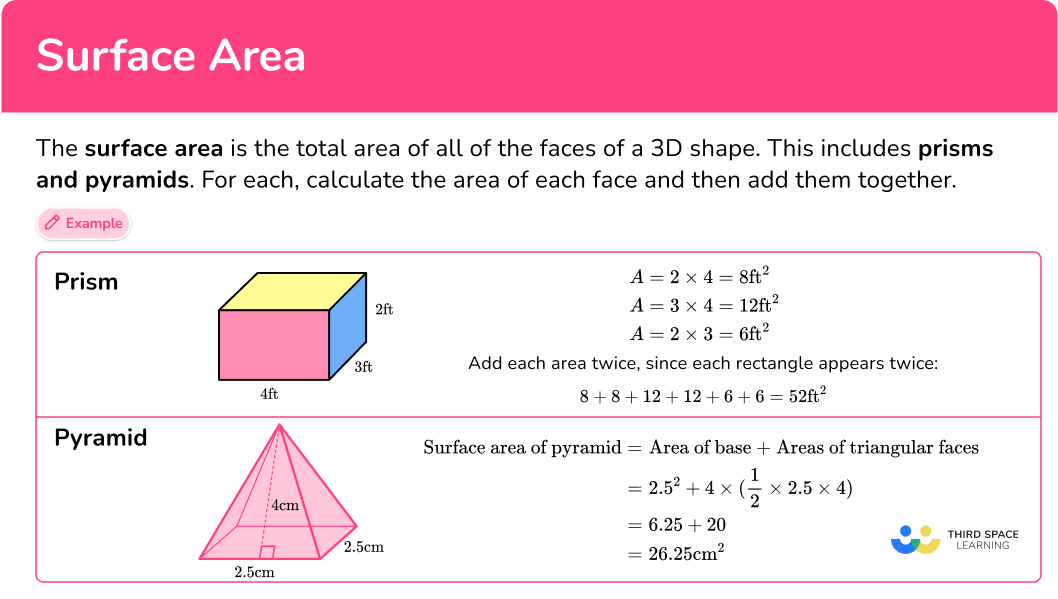## Common Core State Standards

How does this relate to 6 th grade math?

• Grade 6 – Geometry (6.G.A.4)
Represent three-dimensional figures using nets made up of rectangles and triangles, and use the nets to find the surface area of these figures. Apply these techniques in the context of solving real-world and mathematical problems.

## How to calculate the surface area of a prism

In order to calculate the surface area:

1. Calculate the area of each face.
2. Add the area of each face together.
3. Include the units.

## Surface area examples

### Example 1: surface area of a rectangular prism

Calculate the surface area of the rectangular prism.

1. Calculate the area of each face.

A rectangular prism has 6 faces, with 3 pairs of identical faces.

2Add the area of each face together.

Total surface area: 14+14+21+21+6+6=82

OR

Since opposite rectangles are always congruent, you can also use multiplication to solve:

Total surface area: 14 \times 2+21 \times 2+6 \times 2=82

3Include the units.

The measurements on this prism are in m , so the total surface area of the prism is 82 \mathrm{~m}^2.

### Example 2: surface area of a triangular prism with an equilateral triangle – using a net

Calculate the surface area of the triangular prism. The base of the prism is an equilateral triangle with a perimeter of 16.5 \, ft.

First, use the perimeter of the base to find the length of each side. Since an equilateral triangle has all equal sides, s , the perimeter is s+s+s=16.5.

s=5.5 \, ft

You can unfold the triangular prism, and use the net to find the area of each face:

Remember that the edges in a prism are always equal, so if you were to fold up the net, the 5.5 \, ft side of the triangle would combine to form an edge with each corresponding rectangle – making their lengths equal.

The area of each triangular base:

\cfrac{1}{2} \times 4.8 \times 5.5=13.2

The area of each rectangular lateral face:

10 \times 5.5=55

If you have trouble keeping track of all the calculations, use a net:

The area of the base is always equal to the area of the opposite base, in this case the triangles.

Notice, since the triangle is equilateral, all the rectangular faces are equal as well.

Total surface area: 13.2+13.2+55+55+55=191.4

The measurements on this prism are in ft , so the total surface area of the prism is 191.4 \mathrm{~ft}^2.

### Example 3: surface area of a square-based pyramid in cm

All the lateral faces of the pyramid are congruent. Calculate the surface area.

The base is a square with the area 6\times{6}=36\text{~cm}^2.

All four triangular faces are identical, so calculate the area of one triangle, and then multiply the area by 4 .

\begin{aligned} A&= \cfrac{1}{2} \, \times{b}\times{h}\\\\ &=\cfrac{1}{2} \, \times{10}\times{6}\\\\ &=30 \end{aligned}

30\times{4}=120

Add the area of the base and the area of the four triangles:

SA=36+120=156

The side lengths are measured in centimeters, so the area is measured in square centimeters.

SA=156\text{~cm}^{2}

### Example 4: surface area of a rectangular prism – using a net

Calculate the lateral surface area of the rectangular prism. The base of the prism is a square and one side of the base measures 3 \, \cfrac{2}{3} inches.

You can unfold the rectangular prism, and use the net to find the area of each face:

Remember that the edges in a prism are always equal, so if you were to fold up the net, the 3 \cfrac{2}{3} \mathrm{~ft} side of the square would combine to form an edge with each corresponding rectangle – making their lengths equal.

The area of each rectangular lateral face:

\begin{aligned} & 9 \cfrac{4}{5} \, \times 3 \, \cfrac{2}{3} \\\\ &= \cfrac{49}{5} \, \times \cfrac{11}{3} \\\\ &= \cfrac{539}{15} \\\\ &= 35 \, \cfrac{14}{15} \end{aligned}

Remember, you are only finding the area of the lateral faces, so you do not need to calculate the area of the bases.

If you have trouble keeping track of all the calculations, use a net:

Notice, since the square has all equal sides, all the rectangular faces are equal as well.

Total lateral surface area:

\begin{aligned} & 35 \, \cfrac{14}{15}+35 \, \cfrac{14}{15}+35 \, \cfrac{14}{15}+35 \, \cfrac{14}{15} \\\\ & =140 \, \cfrac{56}{15} \\\\ & =143 \, \cfrac{11}{15} \end{aligned}

OR

Since all the lateral faces are congruent, you can also use multiplication to solve:

\begin{aligned} & 4 \times 35 \, \cfrac{14}{15} \\\\ & =\cfrac{4}{1} \, \times \, \cfrac{539}{15} \\\\ & =\cfrac{2,156}{15} \\\\ & =143 \, \cfrac{11}{15} \end{aligned}

The measurements on this prism are in inches, so the total lateral surface area of the prism is 143 \, \cfrac{11}{15} \text {~inches }^2.

### Example 5: surface area of a parallelogram prism with different units

Calculate the surface area of the parallelogram prism.

A parallelogram prism has 6 faces and, like a rectangular prism, it has 3 pairs of identical faces. The base is a parallelogram and all of the lateral faces are rectangular.

In this example, some of the measurements are in cm and some are in m . You must convert the units so that they are the same. Convert all the units to meters (m)\text{: } 40 {~cm}=0.4 {~m} and 50 {~cm}=0.5 {~m}.

Total surface area: 0.48+0.48+1.8+1.8+0.75+0.75=6.06

The measurements that we have used are in m so the surface area of the prism is 6.06 \mathrm{~m}^2.

### Example 6: surface area of a square pyramid – word problem

Mara is making a square pyramid out of cardboard. She cut out 4 acute triangles that have a base of 5 inches and a height of 7.4 inches. How much cardboard will she need to complete the entire square pyramid?

The lateral faces are all congruent, acute triangles.

\begin{aligned} \text {Area of triangle } & =\cfrac{1}{2} \, \times 5 \times 7.4 \\\\ & =18.5 \end{aligned}

Since it is a square pyramid, the base is a square. Each side of the square shares an edge with the base of the triangle, so each side of the square is 5 .

\begin{aligned} \text { Area of square } & =5 \times 5 \\\\ & =25 \end{aligned}

There is one square base and 4 congruent lateral triangular faces.

Total surface area: 25+18.5 \times 4=25+74=99

The measurements on this prism are in inches, so the total surface area of the prism is 99 \text {~inches}^2.

### Teaching tips for the surface area of a prism

• Make sure that students have had time to work with physical 3D models and nets before doing activities that involve finding the surface area of pyramids and prisms.

• Choose worksheets that offer a variety of question types – a mixture of showing the full pyramid or prism versus showing the net, a mixture of solving for the missing surface area versus a missing dimension, and one that includes some word problems.

### Easy mistakes to make

• Calculating volume instead of surface area
Volume and surface area are different things – volume tells us the space within the shape whereas surface area is the total area of the faces.
Particularly with rectangular prisms, it is easy to confuse these two topics if a student does not have a complete understanding of the difference.
For example,

Surface Area: Volume:
Left/right side: 4 \times 5=20 \mathrm{~ft}^2 17 \times 4 \times 5=340 \, f t^3
Front/back: 17 \times 5=85 \mathrm{~ft}^2
Top/bottom: 17 \times 4=68 \mathrm{~ft}^2
20+20+85+85+68+68=346 \mathrm{~ft}^2

• Confusing the base and the height of the prism
No matter how the prism is oriented, the height of a prism is always the side length of the lateral face that does not correspond with the base. The base is always the polygon in which the prism is named after.
For example,
This is a trapezoidal prism. It has a trapezoid as its base. Even though the trapezoids are shown in the front and back position in this image, the trapezoid is still the base and the rectangular sides are the lateral faces.

• Calculating with different units
You need to make sure all measurements are in the same units before calculating surface area.
For example,
Before calculating the surface area, the units need to all be the same. Either the cm needs to be converted to mm or the mm converted to cm.

• Confusing lateral area with total surface area
Lateral area is the area of each of the sides, and total surface area is the area of the bases plus the area of the sides. When asked to find the lateral area, be sure to only add up the area of the sides – which are always rectangles in right prisms (the types of prisms shown on this page).

Note: In oblique prisms the lateral faces are parallelograms.

### Practice surface area of a prism questions

1) The pyramid is composed of four congruent equilateral triangles. Find the surface area of the pyramid.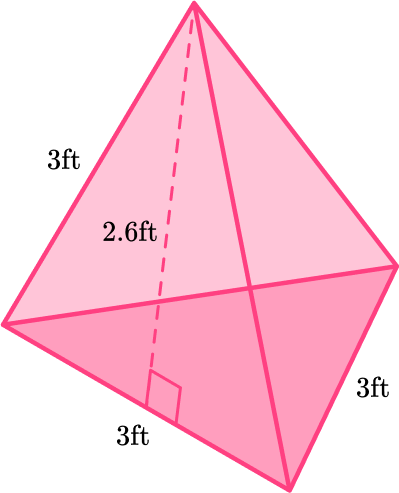70.2 \mathrm{~ft}^231.2 {~ft}^215.6 {~ft}^244.25 {~ft}^2\begin{aligned} \text {Surface area of pyramid }&= \text { Area of base and faces} \\ & \quad \text{ (4 congruent triangles) } \\\\ & =4 \times\left(\cfrac{1}{2} \, \times 3 \times 2.6\right) \\\\ & =4 \times 3.9 \\\\ & =15.6 \mathrm{~ft}^2 \end{aligned}

2) Calculate the surface area of the triangular prism: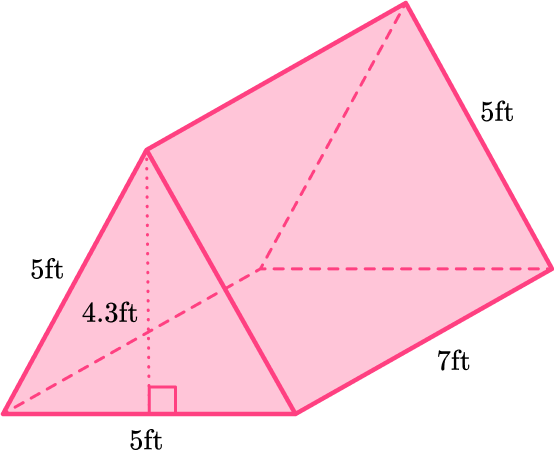\text { 150. } 5 \mathrm{~ft}^278 \mathrm{~ft}^2148 \mathrm{~ft}^2\text { 126. } 5 \mathrm{~ft}^2You can unfold the triangular prism, and use the net to find the area of each face.

Remember that the edges in a prism always fold up together to form the prism – making their lengths equal.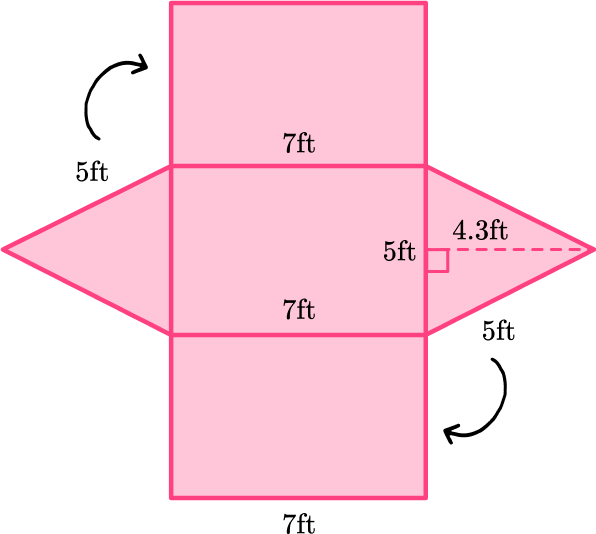The area of each triangular base:

\cfrac{1}{2} \times 5 \times 4.3=10.75

The area of each rectangular lateral face:

7 \times 5=35

If you have trouble keeping track of all the calculations, use a net: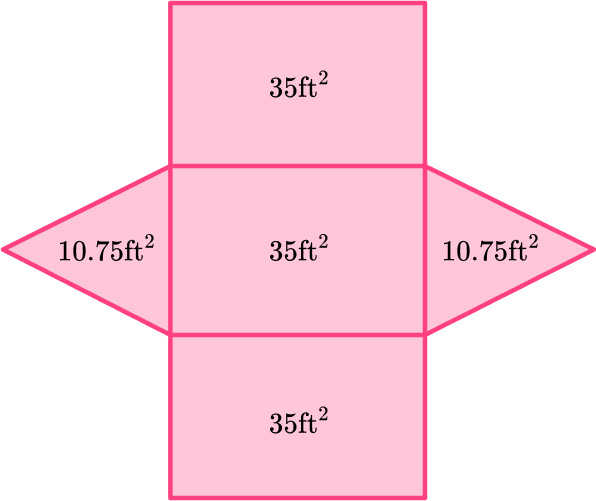Total surface area: 10.75+10.75+35+35+35=126.5 \mathrm{~ft}^2

3) Calculate the surface area of the rectangular prism: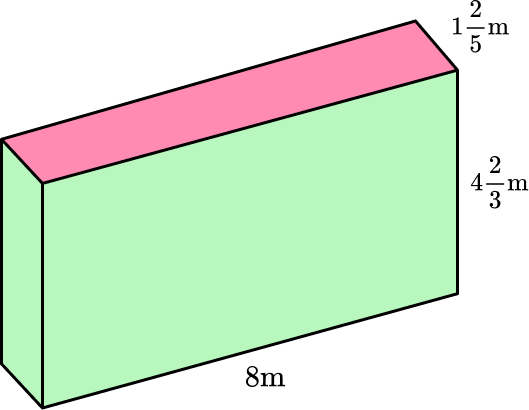110 \, \cfrac{2}{15} {~m}^235 \, \cfrac{7}{15} {~m}^229 \, \cfrac{13}{15} {~m}^2103 \, \cfrac{11}{15} {~m}^2You can unfold the rectangular prism, and use the net to find the area of each face.

Remember that the edges in a prism always fold up together to form the prism – making their lengths equal.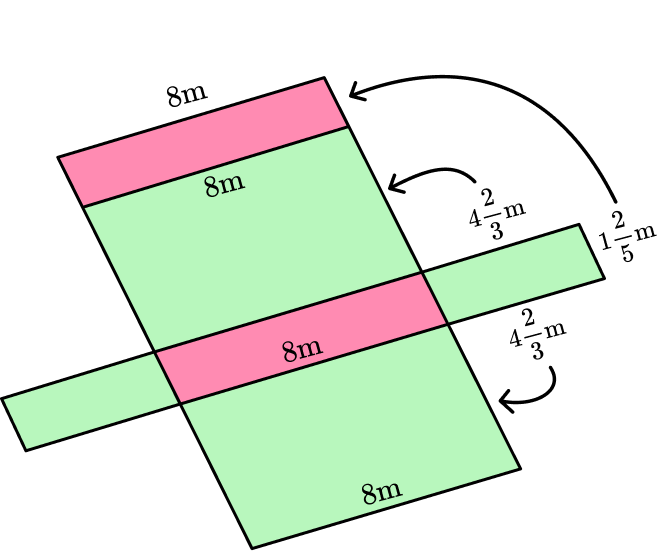The area of each rectangular base:

\begin{aligned} & 1 \cfrac{2}{5} \, \times 8 \\\\ &= \cfrac{7}{5} \, \times \cfrac{8}{1} \\\\ &= \cfrac{56}{5} \\\\ &= 11 \, \cfrac{1}{5} \end{aligned}

The area of each rectangular lateral face:

\begin{aligned} & 4 \, \cfrac{2}{3} \, \times 8 \\\\ &= \cfrac{14}{3} \, \times \cfrac{8}{1} \\\\ &= \cfrac{112}{3} \\\\ &= 37 \, \cfrac{1}{3} \end{aligned}

\begin{aligned} & 4 \, \cfrac{2}{3} \, \times 1 \cfrac{2}{5} \\\\ &= \cfrac{14}{3} \, \times \cfrac{7}{5} \\\\ &= \cfrac{98}{15} \\\\ &= 6 \cfrac{8}{15} \end{aligned}

If you have trouble keeping track of all the calculations, use a net: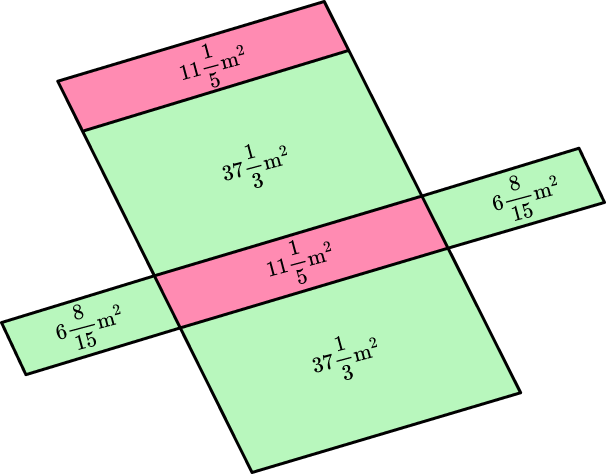Total surface area:

\begin{aligned} & 6 \, \cfrac{8}{15} \, +6 \, \cfrac{8}{15} \, +11 \, \cfrac{1}{5} \, +11 \, \cfrac{1}{5} \, +37 \, \cfrac{1}{3} \, +37 \, \cfrac{1}{3} \\\\ & =6 \, \cfrac{8}{15} \, +6 \, \cfrac{8}{15} \, +11 \, \cfrac{5}{15} , +11 \, \cfrac{5}{15} \, +37 \, \cfrac{3}{15} \, +37 \, \cfrac{3}{15} \\\\ & =108 \, \cfrac{32}{15} \\\\ & =110 \, \cfrac{2}{15} \mathrm{~m}^2 \end{aligned}

4) Here is a net of a square pyramid. Calculate the surface area.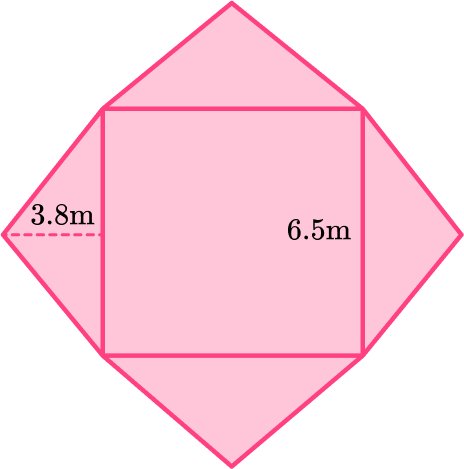54.6 \mathrm{~m}^291.65 \mathrm{~m}^266.95 \mathrm{~m}^2141.05 \mathrm{~m}^2Calculate the area of each face.

The lateral faces are all congruent, acute triangles.

\begin{aligned} \text {Area of triangle } & =\cfrac{1}{2} \, \times 6.5 \times 3.8 \\\\ & =12.35 \end{aligned}

Since it is a square pyramid, the base is a square.

\begin{aligned} \text {Area of square } & =6.5 \times 6.5 \\\\ & =42.25 \end{aligned}

Total surface area = 12.35+12.35+12.35+12.35+42.25=91.65 \mathrm{~m}^2

5) Calculate the surface area of the prism.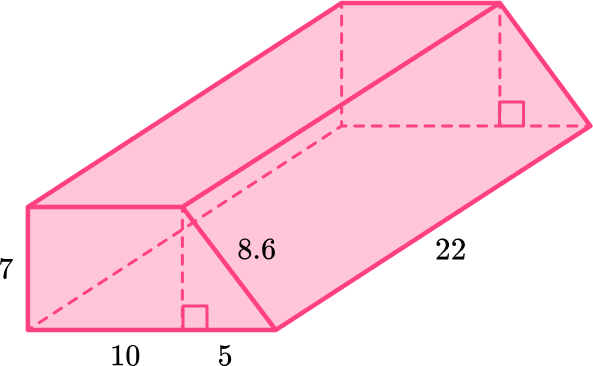2,310 \text { units}^21,068.2 \text { units}^21,033.5 \text { units}^21,560.6 \text { units}^2Calculate the area of each face.

The congruent bases (front and back faces) are composed of a rectangle and a right triangle.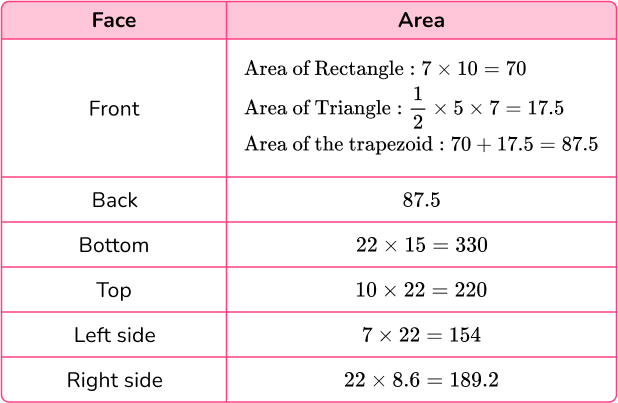Total surface area = 87.5+87.5+330+220+154+189.2=1,068 .2 \text { units}^2

6) Malika was painting the hexagonal prism below. It took 140.8 \text { inches}^2 to cover the entire shape. If the area of the base is \text {10.4 inches}^2 and each side of the hexagon is 2 \text { inches} , what is the height of the prism?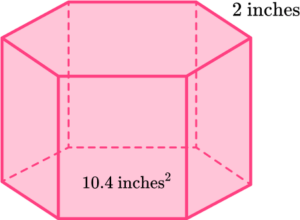120 inches9 inches8 inches10 inchesYou can unfold the hexagonal prism, and use the net to find the area of each face: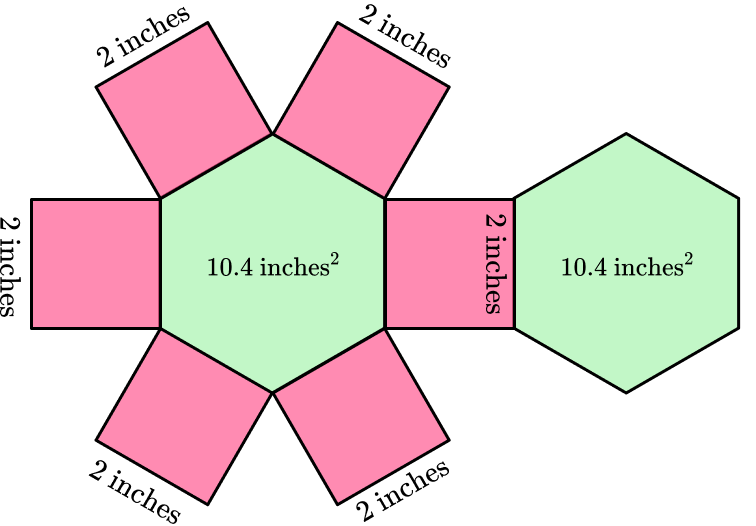Total area of the bases: 10.4+10.4=20.8

Subtract the area of the bases from the total amount of paint Malika used, to see how much was used on the lateral faces:

140.8-20.8=120

The total area of the faces left is 120 \text { inches}^2.

Since the faces are congruent, the total for each face can be found by dividing by 6\text{:}

120 \div 6=20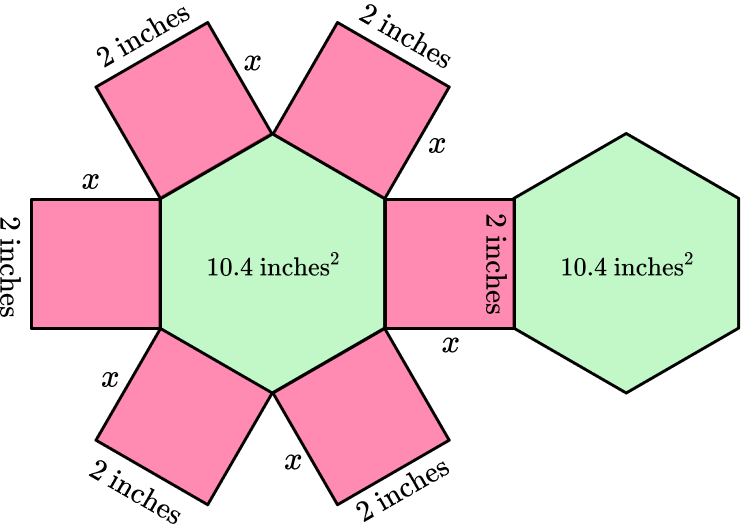Labeling the missing length as x , means the area of each face can be written as 2 \times x or 2 x .

Since each face has an area of 20 \text{ inches}^2 , the missing height can be found with the equation: 2 x=20.

Since 2 \times 10=20 , the missing height is 10 inches.

## Surface area FAQs

What is a cuboid?

A cuboid is a prism with a rectangular base and rectangular lateral sides. It is also known as a rectangular prism.

Are there surface area formulas for prisms?

Some shapes do have a general formula that you can use. For example, the surface area of a rectangular prism uses the formula 2 \: (l b+b h+l h) . There are other formulas, but for all prisms, the general formula is \text {area of } 2 \text { bases }+ \text {area of all lateral faces} .

How do you calculate the surface area of a cube?

Since all the faces have the same area, find the area of the square base and multiply it by 6 .

How do you calculate the surface area of a cylinder?

The surface area of a cylinder is the area of a circle (the two congruent bases) plus the the curved surface area (2 \pi r h).

How do you calculate the surface area of a sphere?

To find the curved surface area, square the radius of the sphere and multiply it by 4 \pi .

## Still stuck?

At Third Space Learning, we specialize in helping teachers and school leaders to provide personalized math support for more of their students through high-quality, online one-on-one math tutoring delivered by subject experts.

Each week, our tutors support thousands of students who are at risk of not meeting their grade-level expectations, and help accelerate their progress and boost their confidence.